# 准确率99.9%！如何用深度学习最快找出放倒的那张X光胸片（代码+数据）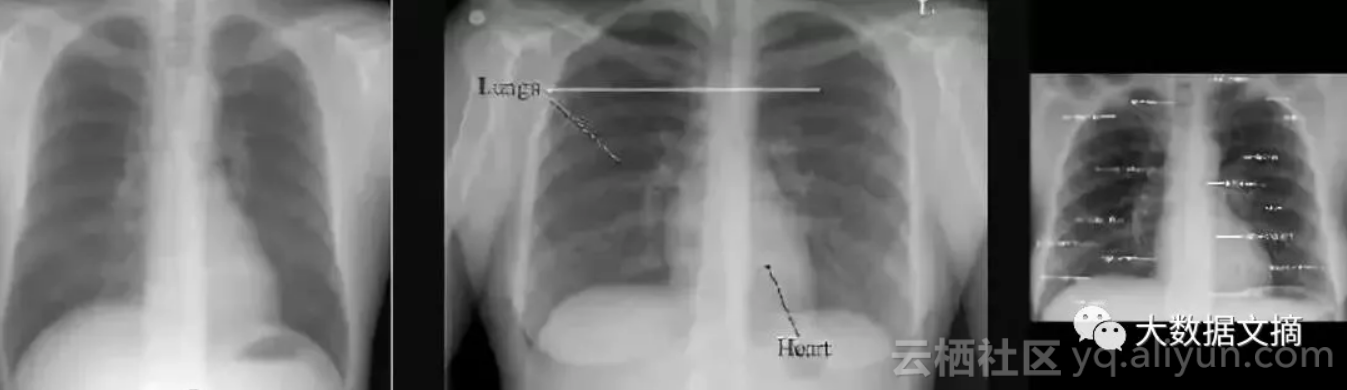https://nihcc.app.box.com/v/ChestXray-NIHCC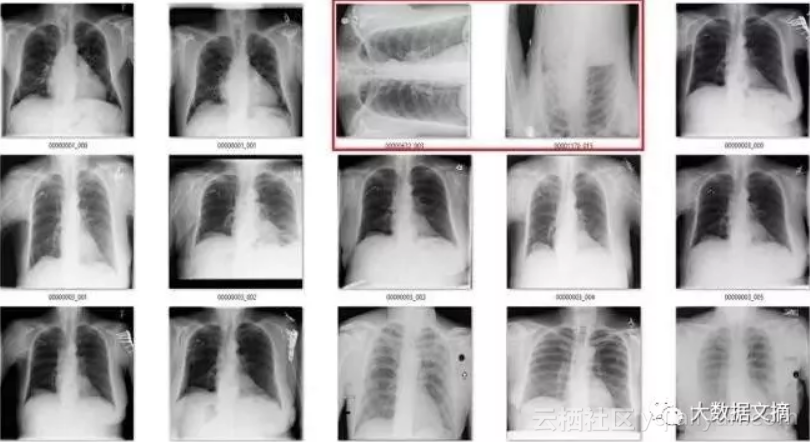def rotate(image):

rotated_image = np.rot90(image, k = np.random.choice(range(1,4)), axes = (1,2))

return rotated_image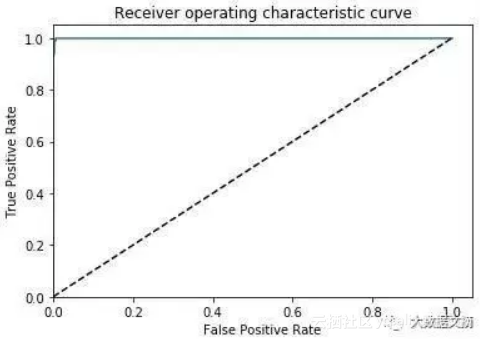AUC=0.999，ACC=0.996，PREC=0.998，REC=0.994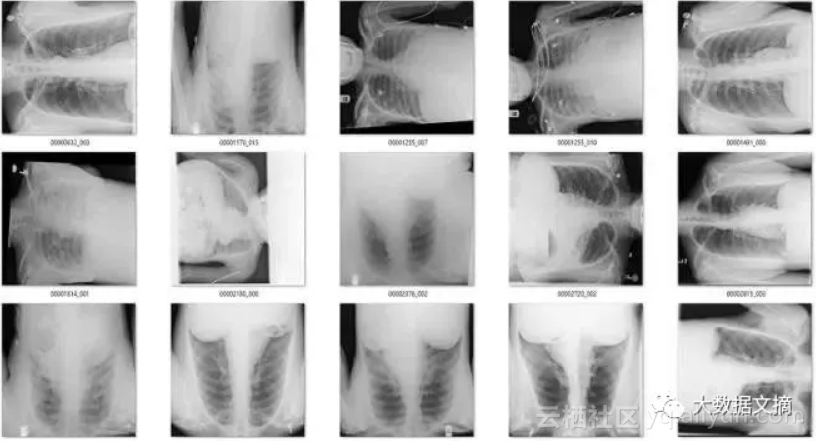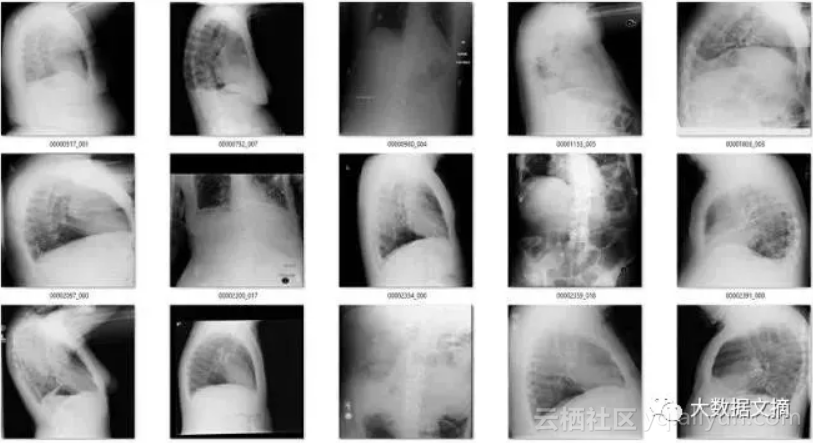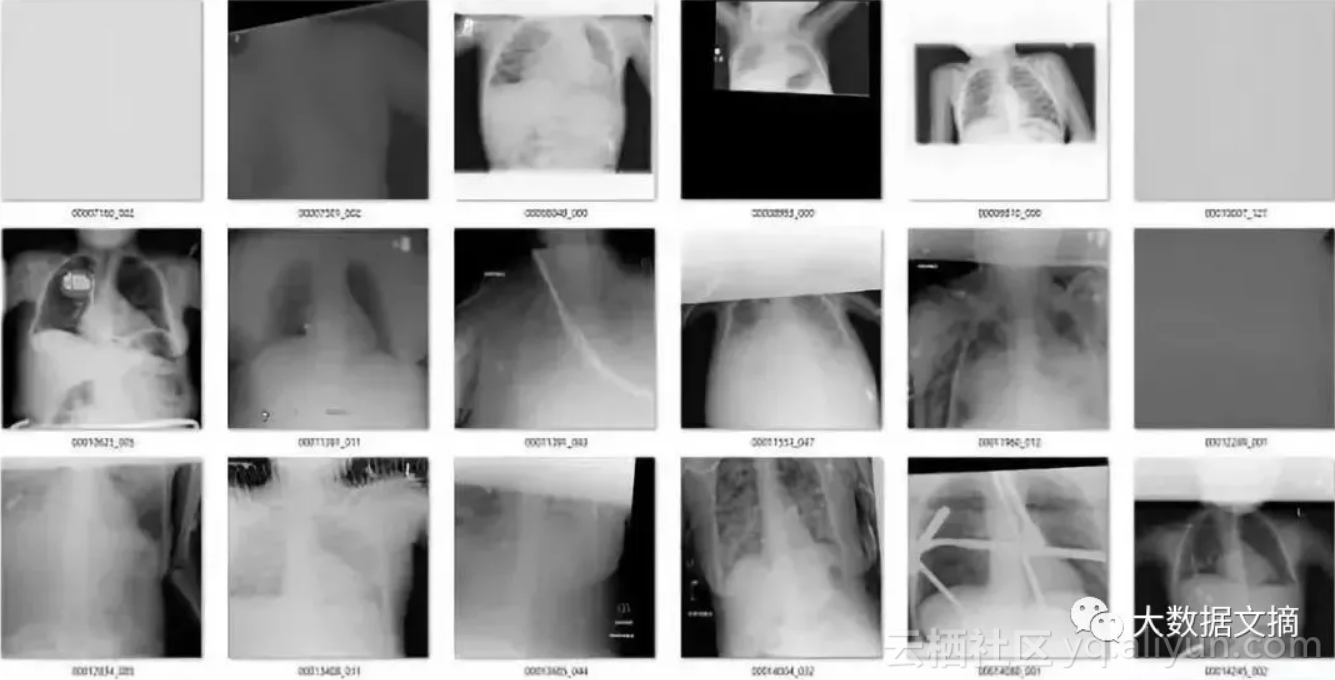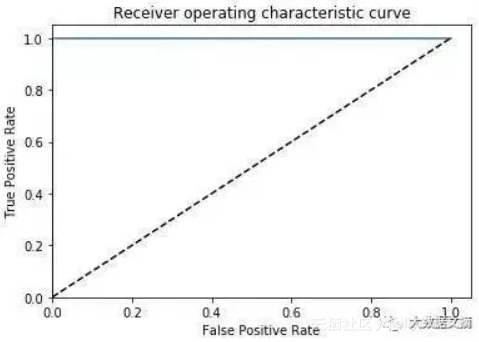AUC=1.0，ACC=0.9995，PREC=0.999，REC=1.0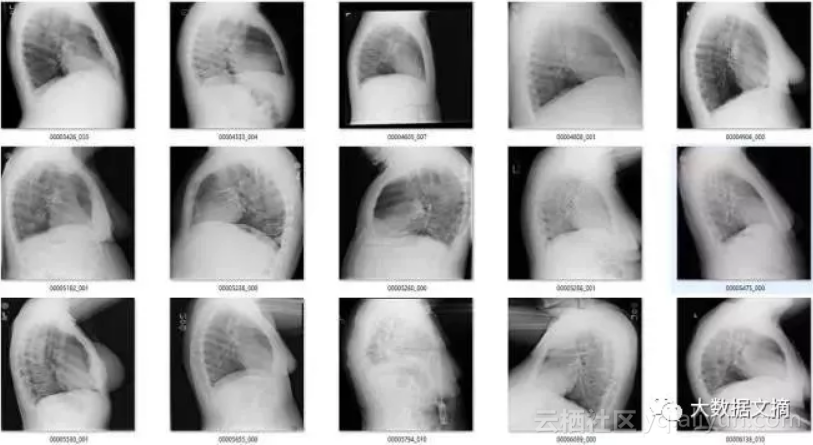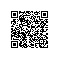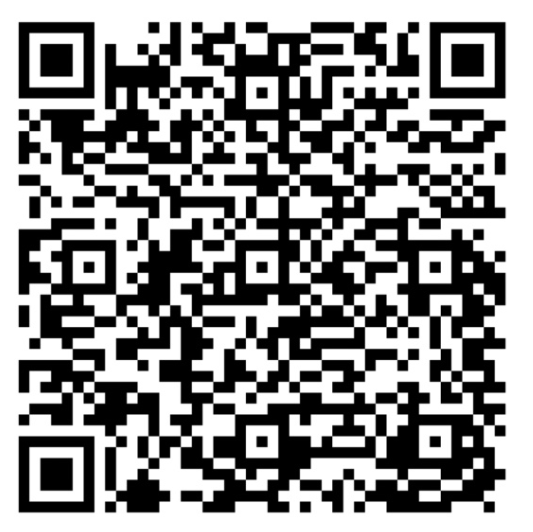使用钉钉扫一扫加入圈子
+ 订阅